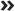Chapter 16

# Testing One Group

IN THIS CHAPTERConducting inferential testsRunning the chi-square goodness of fit testRunning a one-sample t-test

In Chapter 14, we discuss the concept of level of measurement and how to obtain summary statistics for both categorical and continuous variables. In Chapter 15, we talk about the notions of sampling and hypothesis testing. In this chapter, we tie together these ideas and cover two simple inferential tests that can be performed on a single variable: the chi-square goodness of fit test, which is used on categorical variables, and the one-sample t-test, which is used on continuous variables.

## Conducting Inferential Tests

As we mention in Chapter 14, obtaining descriptive statistics can be important for various reasons. For example, descriptive statistics forms the basis for more complex analyses. When you run a chi-square test of independence (see Chapter 17), an independent samples t-test (see Chapter 18), or a simple linear regression (see Chapter 19), you're simply plugging descriptive statistics into a fancy formula and adding the inference that you learned in the previous chapter, which then provides useful information.

Inferential ...

Get SPSS Statistics For Dummies, 4th Edition now with the O’Reilly learning platform.

O’Reilly members experience books, live events, courses curated by job role, and more from O’Reilly and nearly 200 top publishers.